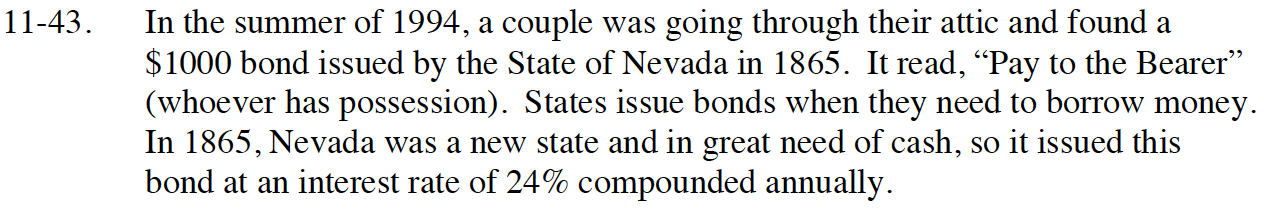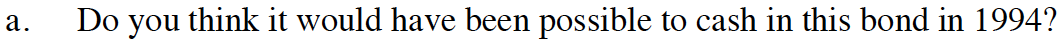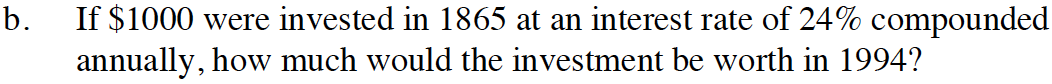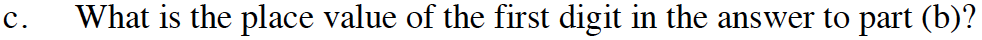### Home > INT3 > Chapter Ch11 > Lesson 11.1.4 > Problem11-43

11-43.
1. In the summer of 1994, a couple was going through their attic and found a $1000 bond issued by the State of Nevada in 1865. It read, “Pay to the Bearer” (whoever has possession). States issue bonds when they need to borrow money. In 1865, Nevada was a new state and in great need of cash, so it issued this bond at an interest rate of 24% compounded annually. Homework Help ✎ 1. Do you think it would have been possible to cash in this bond in 1994? 2. If$1000 were invested in 1865 at an interest rate of 24% compounded annually, how much would the investment be worth in 1994?

3. What is the place value of the first digit in the answer to part (b)?

4. Would the amount be significantly different if it were compounded continuously instead?How many years has it been since the bond was issued? Do you think the state could pay that much?Use A = P(1 + r)n to find the worth of the investment.

A = 1,000 r = 0.24 n = 129

The investment is worth about $1.126 · 1015.The first digit is in the quadrillion place.A ≈$2.791 · 1016, or about 2.68 · 1016 dollars more.